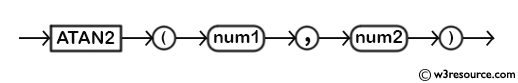# MySQL ATAN2() function

## ATAN2() function

MySQL ATAN2() returns the arc tangent of two numbers for a point on a Cartesian plane.The reverse function is TAN().

This function is useful in -

• It is essential for dealing with coordinate systems, especially when converting between Cartesian (x, y) and polar (r, θ) coordinates.
• ATAN2() helps in calculating slopes and gradients of lines or curves in two-dimensional space.
• It is used in various engineering and physics applications for tasks like angle measurement, motion control, and trajectory planning.
• ATAN2() is used in control systems engineering to design controllers for dynamic systems, as it provides a means to calculate angles and directions of motion.
• In astronomy and satellite tracking systems, ATAN2() is employed to calculate the required angle adjustments for accurately tracking celestial bodies.

Syntax:

```ATAN2(num1, num2);
```

Arguments:

Name Description
num1 A number.
num2 A number.

Syntax Diagram:MySQL Version: 8.0

Example:

Code:

``````SELECT ATAN2(-3,2);
```
```

Explanation:

The above MySQL statement will return the arc tangent in radians of -3 and 2 for a point in a Cartesian plane.

Output:

```mysql> SELECT ATAN2(-3,2);
+--------------------+
| ATAN2(-3,2)        |
+--------------------+
| -0.982793723247329 |
+--------------------+
1 row in set (0.01 sec)
```

All Mathematical Functions

Previous: ASIN()
Next: ATAN()

﻿